# CLASS 11 MATHS CHAPTER-8 BINOMIAL THEOREM

### Expand each of the expression in Exercises 1 to 5.

Question1. Expand the expression (1– 2x)5

Solution :
Using Binomial Theorem,

Question2.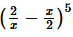Solution :
Using Binomial Theorem,

Question3. Expand the expression (2x – 3)

Solution :
Using Binomial Theorem,

Question4.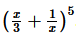Solution :
Using Binomial Theorem,

Question5.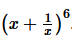Solution :
Using Binomial Theorem,

Using binomial theorem evaluate each of the following:

Question6. Using Binomial Theorem, evaluate (96)3

Solution :
Given: 963

96 = 100 – 4

Using Binomial Theorem,

(96)3 = (100−4)3 = 3C0(100)33C1(100)2(4) +3C2(100)(4)23C3(4)3

=1000000−3(10000)(4)+3(100)(16)−64

=1000000−120000+4800−64

=884736

Question 7 .Using Binomial Theorem, evaluate (102)5

Solution :
Given: (102)5

Using Binomial Theorem,

= (102)5  = (100+2)5 = 5C0(100)5 + 5C1(100)4 (2) + 5C2 (100)3(2)5C3(100)2(2)3 + C4(100)(2)4 + 5C5(2)5

=10000000000+5(100000000)(2)+10(1000000)(4)+10(10000)(8)+5(100)(16)+32

= 10000000000 + 1000000000 + 40000000 + 800000 + 8000 + 32

= 11040808032

Question8. Using Binomial Theorem, evaluate (101)4

Solution :
Given: (101)4

Using Binomial Theorem,

101 = 100+1

(101)4=(100+1)4

=4C0(100)4+4C1(100)3(1)+4C2(100)2(1)2+4C3 (100)(1)3+4C4(1)4

=100000000+4(1000000)+6(10000)+4(100)+1

= 100000000 + 4000000 + 60000 + 400 + 1

= 104060401

9. Using Binomial Theorem, evaluate (99)5

Solution :
Given: (99)5

Using Binomial Theorem,

(99)5=(100−1)5

=5C0(100)55C1(100)4(1)+5C2(100)3(1)25C3(100)(1)3+5C4(100)(1)45C5(1)5

=10000000000−5(100000000)−10(1000000)−10(10000)+5(100)−1=

= 10000000000 – 500000000 + 10000000 – 100000 + 500 – 1

= 9509900499

Question10. Using Binomial Theorem, indicate which number is larger (1.1)10000 or 1000.

Solution :
Given:  (1.1)10000 or 1000.

Using Binomial Theorem,

By splitting 1.1 and then applying Binomial Theorem, the first few terms of (1.1)10000

be obtained as

(1.1)10000=(1+0.1)10000

=10000C0+10000C1(1.1) + Other positive terms

=1+10000×1.1+Other positive terms

=1+11000+Other positive terms>1000

Hence, (1.1)10000>1000

Question11. Find (a + b)4 – (a – b)4. Hence, evaluate(√3 + √2)4– (√3 – √2)4.

Solution :
Given: (a + b)4 – (a – b)4

Using Binomial Theorem,

Question12. Find (x + 1)4 – (x – 1)4  Hence or otherwise evaluate (√ 2 + 1 )6 + (6 -1 )6

Solution :
Given: (x + 1)4 – (x – 1)4

Using Binomial Theorem,

Question13. Show that 9 n+1 is divisible by 64 whenever n is a positive integer.

Solution :
Given:   9 n+1 is divisible by 64

In order to show that 9n+1 −8n−9 is divisible by 64, it has to be prove that, 9n+1−8n−9=64k

, where k is some natural number.

Using Binomial Theorem,

Question14. Prove that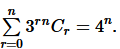Solution :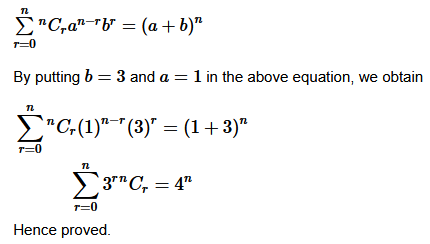### Find the coefficient of:

Question1. Find the coefficient of x5 in (x + 3)8

Solution :It is known that (r+1)th  term, (Tr+1). in the binomial expansion of (a+b)n  is given by Tr+1 nCran−r br

Assuming that x2
occurs in the (r+1)n term of the expansion (x+3)8, we obtain Tr+1 = aCr(x)8-r(3)r

Comparing the indices of x
in x5 in Tr+1. We obtain r = 3 Thus, the coefficient of x5  is 8C3(3)3=8! / 3!5!×33

=(8⋅7⋅6⋅5!  /  3⋅2.5!)∗33=1512

Question2. Find the coefficient of a5b7 in (a – 2b)12

Solution :
General form of the expansion ( r + 1)th is (T r+2)………(i)

Write the general term in the expansion of

Question3. Write the general term in the expansion of (x2 – y)6

Solution :
It is known that the general term Tr+1{ which is the (r+1)term } in the binomial expansion of (a+b)n is given by Tr+1=nCran-rbr.

Thus, the general term in the expansion of (x2−y6)
is Tr+1 = 6Cr(x2)6−r (−y)= (−1)r6 C– x12 −2r⋅yr

Question4. Write the general term in the expansion of (x2 – yx)12, x ≠ 0

Solution :
General form of the expansion Tr+1 is

{ which is the (r+1)th term } in the binomial expansion of (a+b)n is given by Tr+1=nCran−rbr.

Thus, the general term in the expansion of (x2−yx)12
is Tr+1 12Cr(x2)12−t(−yx)r

=(−1)r12Cr−x24−2r−yr

=(−1)r−2Crx24−r⋅yr

Question5. Find the 4th term in the expansion of (x – 2y)12 .

Solution :
It is known (r+1)n term, Tr+1. in the binomial expansion of (a+b)n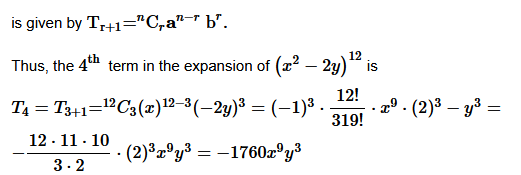Question6. Find the 13th term in the expansion of

Solution :
It is known (r+1)th term, Tr+1, in the binomial expansion of (a+b)n is given by Tε+1 = nCran−1br

Thus, the 13th
term in the expansion of

Find the middle terms in the expansion of:

Question7.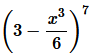Solution :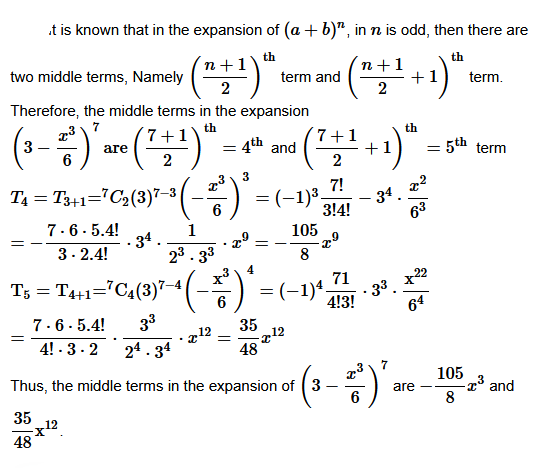Question8. (x/3 + 9y)10

Solution :
Here (a + b )which is an even number.

Therefore, the middle terms are (x/3 + 9y)10 is 6th term.

General form of the expansion

Question9. In the expansion of (1 + a)m + n, prove that coefficients of am and an are equal.

Solution :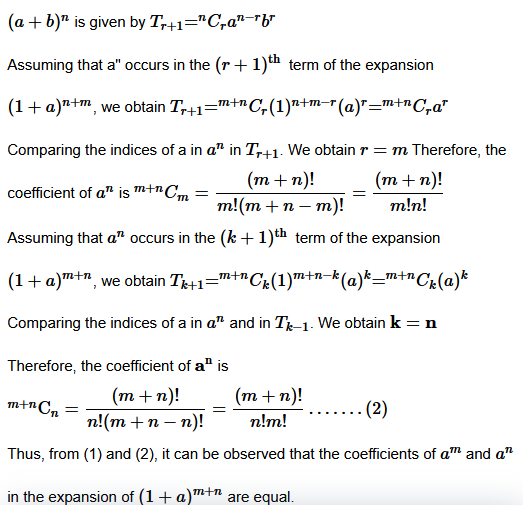Question10. The coefficients of the (r – 1)th, rth and (r + 1)th terms in the expansion of (x + 1)n are in the ratio 1:3:5. Find n and r.

Solution :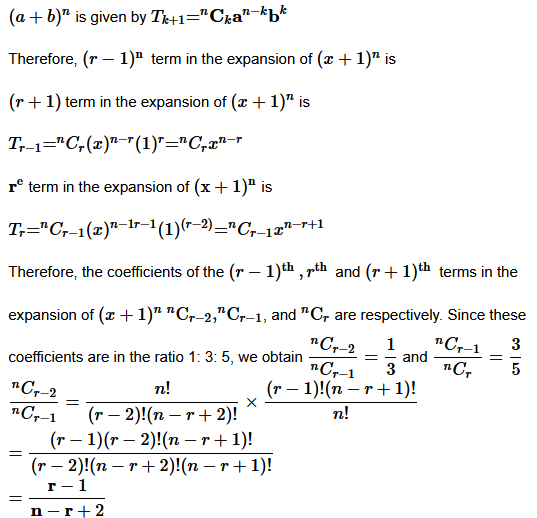Question11.  Prove that the coefficient of xn in the expansion of (1 + x)2n is twice the coefficient of xn in the expansion of (1 + x)2n–1 .

Solution :

= It is known that (r+1)th  term, (Tr+1), in the binomial expansion of (a+b)n is given by Tr+1=nCran−rbr

Assuming that xn
occurs in the (r+1)n term of the expansion of (1+x)2n, we obtain Tr+1=2nCr(1)2n−r(x)r

=2nCr(x)r

Comparing the indices of x
in xn and in Tr+1, we obtain r=n Therefore, the coefficient of xn in the expansion of (1+x)2n is

### Miscellaneous Exercise

Question1. Find a, b and n in the expansion of (a + b)n if the first three terms of the expansion are 729, 7290 and 30375, respectively.

Solution :
Given: (a + b)n

Tr+1 = nCr an-t br

The first three terms of the expansion are given as 729, 7290 and 30375 respectively. Then we have,

T1 = nC0 an-0 b0 = an = 729….. 1

T2 =nC1 an-1 b1 = nan-1 b = 7290…. 2

T3 = nC2 an-2 b2 = {n (n -1)/2 }an-2 b2 = 30375……3

Dividing 2 by 1 we get

Question2. Find a if the coefficients of x2 and x3 in the expansion of (3 + ax)9 are equal.

Solution :

Question3. Find the coefficient of x5 in the product (1 + 2x)6 (1 – x)7 using binomial theorem.

Solution :
Using Binomial Theorem,

Question4. If a and b are distinct integers, prove that a – b is a factor of an – bn, whenever n is a positive integer.

[Hint: write an = (a – b + b)n and expand]

Solution :

n order to prove that (a – b) is a factor of (an – bn), it has to be proved that

an – bn = k (a – b) where k is some natural number.

a can be written as a = a – b + b

an = (a – b + b)n = [(a – b) + b]n

nC0 (a – b)n + nC1 (a – b)n-1 b + …… + n C n bn

a– bn = (a – b) [(a –b)n-1 + nC1 (a – b)n-1 b + …… + bn]

an – bn = (a – b) k

Where k = [(a –b)n-1 nC1 (a – b)n-1 b + …… + n C n bn] is a natural number

Question5. Evaluate: (√3 + √2)– (√3 – √2)6

Solution :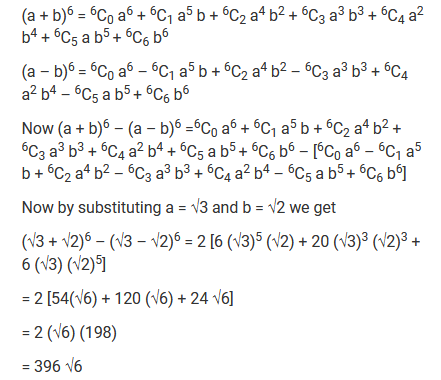Question6. Find the value of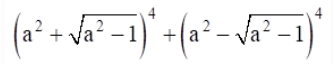Solution :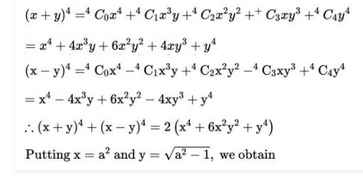Question7. Find an approximation of (0.99)using the first three terms of its expansion.

Solution :
Here .99 can be written as

0.99 = 1 – 0.01

Now by applying binomial theorem we get

(o. 99)5 = (1 – 0.01)5

= 5C0 (1)– 5C1 (1)4 (0.01) + 5C2 (1)3 (0.01)2

= 1 – 5 (0.01) + 10 (0.01)2

= 1 – 0.05 + 0.001

= 0.951

Question8. Find n if the ratio of the fifth term from the beginning to the fifth term from the end in the expansion of is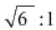Solution :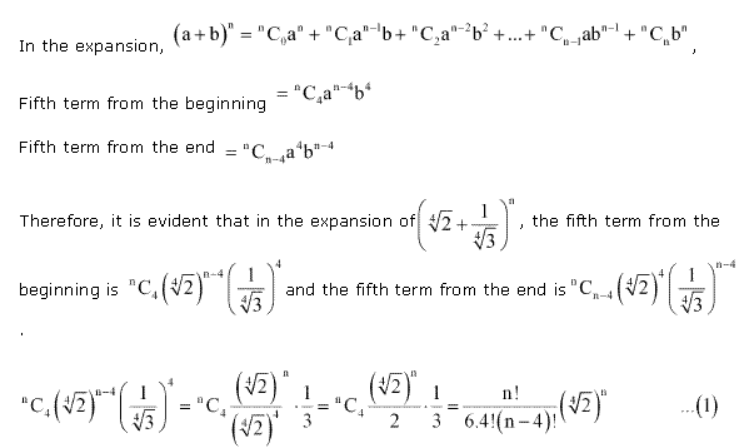Question9. Expand using binomial theorem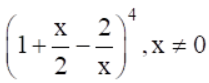Solution :
We have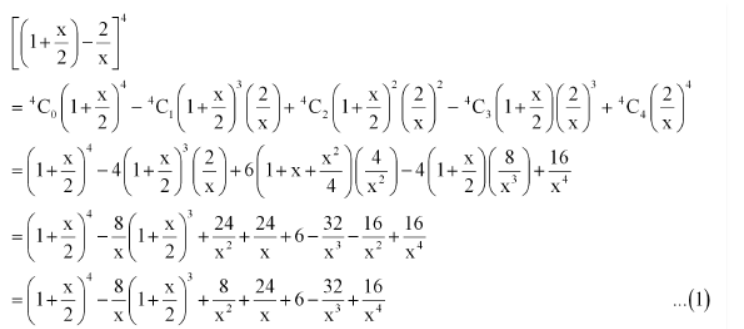Question10. Find the expansion of (3x2 – 2ax + 3a2)3using binomial theorem.

Solution :
Here

=  We know that (a + b)3 = a3 + 3a2b + 3ab2 + b3

Putting a = 3x2 & b = -a (2x-3a), we get
[3x2 + (-a (2x-3a))]3

= (3×2)3+3(3x2)2(-a (2x-3a)) + 3(3x2) (-a (2x-3a))2 + (-a (2x-3a))3

= 27x6 – 27ax4 (2x-3a) + 9a2x2 (2x-3a)2 – a3(2x-3a)3

= 27x6 – 54ax5 + 81a2x4 + 9a2x2 (4x2-12ax+9a2) – a3 [(2x)3 – (3a)3 – 3(2x)2(3a) + 3(2x)(3a)2]

= 27x6 – 54ax5 + 81a2x4 + 36a2x4 – 108a3x3 + 81a4x2 – 8a3x3 + 27a6 + 36a4x2 – 54a5x

= 27×6 – 54ax5+ 117a2x4 – 116a3x3 + 117a4x2 – 54a5x + 27a6

Thus, (3x2 – 2ax + 3a2)3

= 27x6 – 54ax5+ 117a2x4 – 116a3x3 + 117a4x2 – 54a5x + 27a6

Get 30% off your first purchase!

X
error: Content is protected !!
Scroll to Top